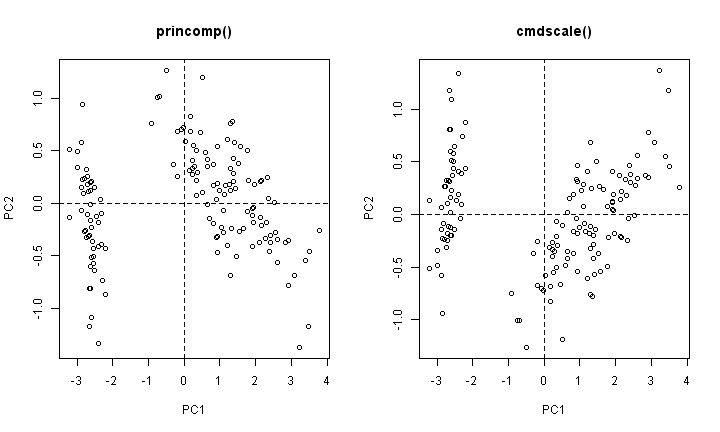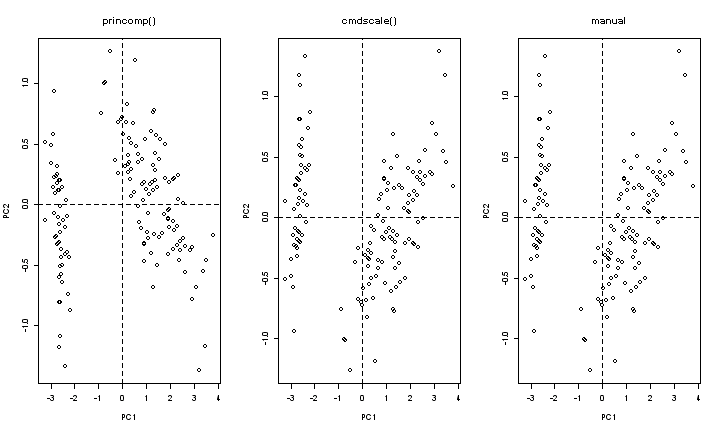## Tuesday, 21 January 2014

### Principal Component Analysis

Principal component are orthogonal axes that best represent the characteristics of given variables. Hence, principal components are often used in multidimensional scaling when performing clustering analysis.

In R, there are number of ways principal components could be derived.

In the base package, princomp() function is probably the easiest way to derive principal components. It is simple to use and is quick. The below example shows how this function is used.

Dat <- iris princomp(Dat[, 1:4])

## Call:
## princomp(x = Dat[, 1:4])
##
## Standard deviations:
## Comp.1 Comp.2 Comp.3 Comp.4
## 2.0494 0.4910 0.2787 0.1539
##
## 4 variables and 150 observations.

The below is an example of using formula.

princomp(~Sepal.Length + Sepal.Width + Petal.Length + Petal.Width, Dat)

## Call:
## princomp(formula = ~Sepal.Length + Sepal.Width + Petal.Length +
## Petal.Width, data = Dat)
##
## Standard deviations:
## Comp.1 Comp.2 Comp.3 Comp.4
## 2.0494 0.4910 0.2787 0.1539
##
## 4 variables and 150 observations.

As it can be seen above, the resulting principal components are same regardless of the way the princomp() function is coded.

As a default, princomp() function converts the data to covariance matrix in order to derive principal components. If the data set has a variable that has 0 (zero) variance (i.e. constant variable), princomp() function treats the variable as its own principal component (i.e. it retains the variable as it is). If you want to use correlation matrix instead, write the code as shown below.

princomp(Dat[, 1:4], cor = TRUE)

## Call:
## princomp(x = Dat[, 1:4], cor = TRUE)
##
## Standard deviations:
## Comp.1 Comp.2 Comp.3 Comp.4
## 1.7084 0.9560 0.3831 0.1439
##
## 4 variables and 150 observations.

It should be noted that correlation matrix produces error if there are any constant variables present. Hence, it is best to remove them before deriving principal components this way.

To extract corresponding principal components axis (i.e. eigen vectors) from the output of princomp(), we look for the attribute called 'loading'.

princomp(Dat[, 1:4])\$loading

##
## Loadings:
## Comp.1 Comp.2 Comp.3 Comp.4
## Sepal.Length 0.361 -0.657 0.582 0.315
## Sepal.Width -0.730 -0.598 -0.320
## Petal.Length 0.857 0.173 -0.480
## Petal.Width 0.358 -0.546 0.754
##
## Comp.1 Comp.2 Comp.3 Comp.4
## SS loadings 1.00 1.00 1.00 1.00
## Proportion Var 0.25 0.25 0.25 0.25
## Cumulative Var 0.25 0.50 0.75 1.00

To find the converted values (i.e. principal components) from the output, we look for the attribute called 'score'.

head(princomp(Dat[, 1:4])\$score)

## Comp.1 Comp.2 Comp.3 Comp.4
## [1,] -2.684 -0.3194 0.02791 0.002262
## [2,] -2.714 0.1770 0.21046 0.099027
## [3,] -2.889 0.1449 -0.01790 0.019968
## [4,] -2.745 0.3183 -0.03156 -0.075576
## [5,] -2.729 -0.3268 -0.09008 -0.061259
## [6,] -2.281 -0.7413 -0.16868 -0.024201

An alternate way to find principal components, subject to satisfying conditions to be described, is using cmdscale() function. This function is also one of base package functions, but is primarily used for multidimensional scaling. The multidimensional scaling based on Euclidean distance should produce the same output as the principal component conversion based on covariance matrix. Hence, the condition for this to be used as an alternate method is that princomp() uses the data converted to covariance matrix, and cmdscale() uses data converted to distance matrix based on Euclidean distance (e.g. use dist() function).

D <- dist(Dat[, 1:4]) head(cmdscale(D, k = 4))

## [,1] [,2] [,3] [,4]
## [1,] -2.684 0.3194 0.02791 0.002262
## [2,] -2.714 -0.1770 0.21046 0.099027
## [3,] -2.889 -0.1449 -0.01790 0.019968
## [4,] -2.745 -0.3183 -0.03156 -0.075576
## [5,] -2.729 0.3268 -0.09008 -0.061259
## [6,] -2.281 0.7413 -0.16868 -0.024201

In cmdscale() function, 'k' represents the desired number of dimensions in the output. The output displays principal component axes (i.e. eigen vectors) in the order of decreasing eigen values, and reduction of dimensions removes the vectors with smallest eigen values first.

The below graph compares the output of princomp() and cmdscale().

par(mfrow = c(1, 2)) plot(princomp(Dat[, 1:4])\$score[, 1:2], main = "princomp()", xlab = "PC1", ylab = "PC2") abline(h = 0, lty = 2) abline(v = 0, lty = 2) plot(cmdscale(dist(Dat[, 1:4]), k = 4)[, 1:2], main = "cmdscale()", xlab = "PC1", ylab = "PC2") abline(h = 0, lty = 2) abline(v = 0, lty = 2)The results are mirror images along x-axis, but are identical as the direction of principal component does not affect the outcome of the analysis.

The third method expands out the conversion mathematically. This is useful if you wish to make modifications during the conversion process.

Firstly, any constant variables are removed.

Dat <- iris[, 1:4]
V <- apply(Dat, 2, var) Input <- Dat[, which(V > 0)]

Secondly, the variables are centered to their respective means.

Mean <- apply(Input, 2, mean) AdjD <- t(apply(Input, 1, function(x) (x - Mean)))

Create covariance matrix. You may use cov() instead of var() which will produce the same result.

CovA <- var(AdjD)

Derive eigen vectors. The parameter 'symmetric' needs to be set to 'TRUE' in order to stop R from incorporating duplicated values.

EigVec <- eigen(CovA, symmetric = TRUE)

The data set is converted to its principal components.

temp <- t(EigVec[]) %*% t(AdjD) ConvD <- t(temp) head(ConvD)

## [,1] [,2] [,3] [,4]
## [1,] -2.684 0.3194 -0.02791 0.002262
## [2,] -2.714 -0.1770 -0.21046 0.099027
## [3,] -2.889 -0.1449 0.01790 0.019968
## [4,] -2.745 -0.3183 0.03156 -0.075576
## [5,] -2.729 0.3268 0.09008 -0.061259
## [6,] -2.281 0.7413 0.16868 -0.024201

The below graph compares the resulting principal components from the above 3 methods.

par(mfrow = c(1, 3)) plot(princomp(Dat[, 1:4])\$score[, 1:2], main = "princomp()", xlab = "PC1", ylab = "PC2") abline(h = 0, lty = 2) abline(v = 0, lty = 2) plot(cmdscale(dist(Dat[, 1:4]), k = 4)[, 1:2], main = "cmdscale()", xlab = "PC1", ylab = "PC2") abline(h = 0, lty = 2) abline(v = 0, lty = 2) plot(ConvD[, 1:2], main = "manual", xlab = "PC1", ylab = "PC2") abline(h = 0, lty = 2) abline(v = 0, lty = 2)